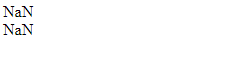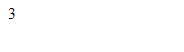# Math.sqrt() Method with Example in JavaScript

JavaScript | Math.sqrt() Method: Here, we are going to learn about the sqrt() method of Math class in JavaScript with Examples.
Submitted by Shivang Yadav, on November 30, 2019

## JavaScript | Math.sqrt() Method

The Math.sqrt() method is inbuilt in JavaScript to find the square root of a number. In this tutorial, we will learn about the sqrt() method with examples.

In the math library inbuilt in the JavaScript programming language. There are many methods that support mathematical operations. The sqrt() method in JavaScript is used to find the square root of the number that is passed to the method as parameters.

Syntax:

```    Math.sqrt(n);
```

Parameter(s):

• n – It takes only one value which is an integer whose square root is to be found.

Return value:

The return type of this method is number, it returns the square root of the given parameter, if the given number is negative then it returns NaN.

Example 1: passing integers to the sqrt() method.

```<!DOCTYPE html>
<html>

<title>Page Title</title>

<body>

<script>
document.write(Math.sqrt(4) + "<br>");
document.write(Math.sqrt(12.56));
</script>

</body>

</html>
```

OutputPassing other values to the sqrt() method

All the following values if passed to the sqrt() method will return NaN. Denoting not a number passed to the method: Negative numeric value, non-numeric strings, an array with multiple numeric values, empty string or array.

Example 2: passing error values to method that will return NaN.

```<!DOCTYPE html>
<html>

<title>Page Title</title>

<body>

<script>
document.write(Math.sqrt(-6) + "<br>");
document.write(Math.sqrt("Include Help") + "<br>");
</script>

</body>

</html>
```

OutputYou can also to operations inside the method and the result of these operations will be passed to the method to calculate the square root.

Example 3: passing expressions to sqrt() method.

```<!DOCTYPE html>
<html>

<title>Page Title</title>

<body>

<script>
document.write(Math.sqrt(5.3 + 3.7) + "<br>");
</script>

</body>

</html>
```

OutputPreparation

What's New

Top Interview Coding Problems/Challenges!